# 1 softmax loss

softmax loss是我们最熟悉的loss之一，在图像分类和分割任务中都被广泛使用。Softmax loss是由softmax和交叉熵(cross-entropy loss)loss组合而成，所以全称是softmax with cross-entropy loss，在caffe，tensorflow等开源框架的实现中，直接将两者放在一个层中，而不是分开不同层，可以让数值计算更加稳定，因为正指数概率可能会有非常大的值。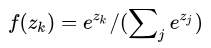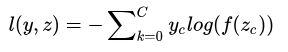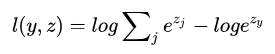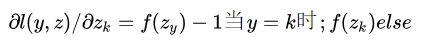``````Dtype* bottom_diff =
bottom->mutable_cpu_diff();
const Dtype* prob_data = prob_.cpu_data();
caffe_copy(prob_.count(), prob_data,
bottom_diff);
const Dtype* label =
bottom->cpu_data();
int dim = prob_.count() / outer_num_;
int count = 0;
for (int i = 0; i < outer_num_; ++i) {
for (int j = 0; j < inner_num_; ++j) {
const int label_value =
static_cast<int>(label[i * inner_num_ + j]);
if (has_ignore_label_ && label_value == ignore_label_) {
for (int c = 0; c <bottom->shape(softmax_axis_); ++c) {
bottom_diff[i * dim + c *inner_num_ + j] = 0;
}
}else
{
bottom_diff[i * dim + label_value * inner_num_ + j] -= 1;
++count;
}
}
}``````

Test_softmax_with_loss_layer.cpp

Forward测试是这样的，定义了个bottom blob data和bottom blob label，给data塞入高斯分布数据，给label塞入0～4。

``````blob_bottom_data_(new Blob<Dtype>(10, 5, 2, 3))
blob_bottom_label_(new Blob<Dtype>(10, 1, 2, 3))``````

``````Dtype accum_loss = 0;
for (int label = 0; label < 5; ++label) {
layer_param.mutable_loss_param()->set_ignore_label(label);
layer.reset(new SoftmaxWithLossLayer<Dtype>(layer_param));
layer->SetUp(this->blob_bottom_vec_,this->blob_top_vec_);
layer->Forward(this->blob_bottom_vec_, this->blob_top_vec_);
accum_loss += this->blob_top_loss_->cpu_data();
}
// Check that each label was included all but once.
EXPECT_NEAR(4 * full_loss, accum_loss, 1e-4);``````

``````TYPED_TEST(SoftmaxWithLossLayerTest,
typedef typename TypeParam::Dtype Dtype;
LayerParameter layer_param;
//labels are in {0, ..., 4}, so we'll ignore about a fifth of them
layer_param.mutable_loss_param()->set_ignore_label(0);
SoftmaxWithLossLayer<Dtype> layer(layer_param);
}``````

# 2 weighted softmax loss【1】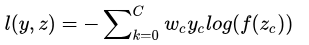wc就是这个权重，像刚才所说，c=0代表边缘像素，c=1代表非边缘像素，则我们可以令w0=1，w1=0.001，即加大边缘像素的权重。

focal loss是针对类别不均衡问题提出的，它可以通过减少易分类样本的权重，使得模型在训练时更专注于难分类的样本，其中就是通过调制系数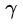来实现。

# 3 soft softmax loss【2】# 4 Large-Margin Softmax Loss【3】

softmax loss擅长于学习类间的信息，因为它采用了类间竞争机制，它只关心对于正确标签预测概率的准确性，忽略了其他非正确标签的差异，导致学习到的特征比较散。文【3】中提出了Large-Margin Softmax Loss，简称为L-Softmax loss。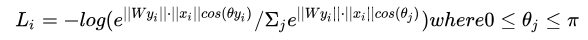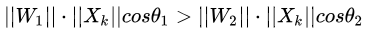L-Softmax loss中，m是一个控制距离的变量，它越大训练会变得越困难，因为类内不可能无限紧凑。

# 6 L2-constrained softmax loss【5】与NormFace【6】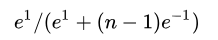# 7 large margin cosine margin【7】

cosine loss中，特征和权值都被归一化。关于cosine loss的解释，在于它相比soft Max loss，L-softmax loss等，更加明确地约束了角度，使得特征更加具有可区分度。

https://github.com/longpeng2008/Caffe_Long

【1】Xie S, Tu Z.Holistically-nested edge detection[C]//Proceedings of the IEEE international conference on computer vision. 2015: 1395-1403.

【2】Hinton G,Vinyals O, Dean J. Distilling the knowledge in a neural network[J]. arXiv
preprint arXiv:1503.02531, 2015.

【3】Liu W, Wen Y,Yu Z, et al. Large-Margin Softmax Loss for Convolutional Neural
Networks[C]//ICML. 2016: 507-516.

【4】Liu W, Wen Y,Yu Z, et al. Sphereface: Deep hypersphere embedding for face
recognition[C]//The IEEE Conference on Computer Vision and Pattern Recognition
(CVPR). 2017, 1.

【5】Ranjan R, Castillo C D, Chellappa R. L2-constrained softmax loss for discriminative face verification[J]. arXiv preprint arXiv:1703.09507, 2017.

【6】Wang F, Xiang X, Cheng J, et al. NormFace: \$ L_2 \$ Hypersphere Embedding for Face Verification[J]. arXiv preprint arXiv:1704.06369, 2017.

【7】Wang H, Wang Y, Zhou Z, et al. CosFace: Large margin cosine loss for deep face recognition[J]. arXiv preprint arXiv:1801.09414, 2018.

【8】Wang F, Liu W,Liu H, et al. Additive Margin Softmax for Face Verification[J]. arXiv preprint arXiv:1801.05599, 2018.

【9】Deng J, Guo J, Zafeiriou S. ArcFace: Additive Angular Margin Loss for Deep Face
Recognition[J]. arXiv preprint arXiv:1801.07698, 2018.

【10】Lin T Y, Goyal P, Girshick R, et al. Focal loss for dense object detection[J]. IEEE transactions on pattern analysis and machine intelligence, 2018.

*延伸阅读

## CVPR 2018 | Repulsion loss：专注于遮挡情况下的行人检测